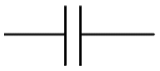#### A PHP Error was encountered

Severity: Warning

Message: count(): Parameter must be an array or an object that implements Countable

Filename: controllers/formulas.php

Line Number: 37

Capacitor Formula Online - Tutorpace

# Capacitor Formula

## Online Tutoring Is The Easiest, Most Cost-Effective Way For Students To Get The Help They Need Whenever They Need It.

A capacitor is made up of two terminal electronic component which stores energy in the form of electric charge. This component consists of two conducting plates which are separated by an insulating material known as dielectric medium. When the potential difference is applied across the conductors, it polarizes the ions to store the charges in the dielectric medium. Figure represents the circuit symbol of a circuit.Capacitance is inversely proportional to the distance between the dielectric plates and directly proportional to the surface are of dielectric plates.

1 µF =10^-6F

1 pF = 10^-12 F

The relation between the capacitance, charge and voltage is given by

C = Q/V

Where C = Capacitance of the capacitor

Q = Charge stored by the capacitor

V = Voltage applied across the capacitor

Example: Find the capacitance of the capacitor if 12 coulomb of charge if flowing and potential applied is 3V.

Solution: Given that,

Charge Q = 12 C and

Voltage applied V = 3 V

Now, we have the formula for capacitance,

C = Q/V

C = 12/3 = 4 F.

Example: If 10 coulomb of charge is flowing and potential applied is 4V, find the capacitance of the capacitor in microfarad.

Solution: Given that,

Charge Q = 10 C and

Voltage applied V = 4 V

Now, we have the formula for capacitance,

C = Q/V

C = 10/4 = 2.5 F = 2.5 x 10^-6 µF.